Tutorial 10 – Encapsulation in Python – Part 1

What you will Learn:
• Public variable
• Private variable
• Getters
• Class variable
• Create object inside the class
• Setters
• Conclusion

Public variable
Create an ‘Engine’ class having 2 methods: __init__ & start.
In the __init__ method we define the capacity of the engine & in the ‘start’ method we are printing the string. We than create an object of this class & print the capacity. Remember that the objects are created outside of the class (line 8)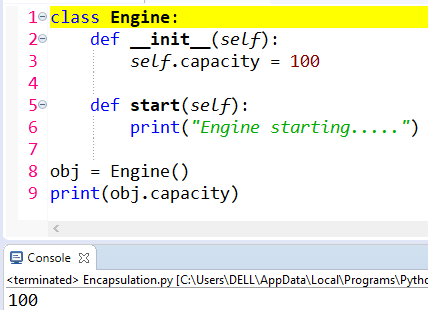Figure 1

Now see below line number 9. The capacity now has the value 123. The console now prints this new value. So basically we have changed the scope of the variable outside class. The reason we were able to change the value of the ‘capacity’ variable is because ‘capacity’ variable is defined as ‘public’ type in line number 3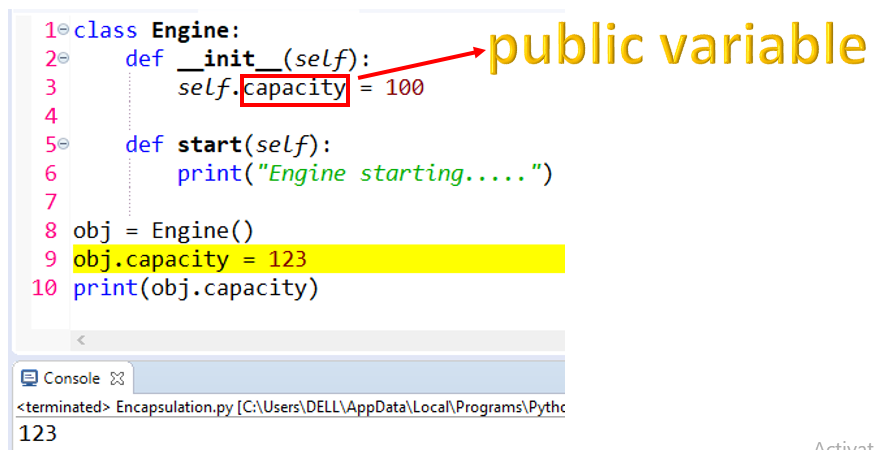Figure 2: public variable

Private variable
What if we don’t want to change the scope of the variable outside class?
To do that, we have to change the scope of the variable from ‘public’ to ‘private’. The private variables are represented in python using double underscore __ (see line 3 below)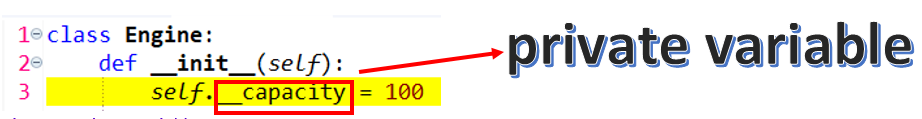Figure 3: private variable

Now comment line number 9 (see below). When we run the program we get an error
“AttributeError: 'Engine' object has no attribute 'capacity'”.
The reason for this error is because the scope of the ‘capacity’ variable is only limited to inside the class. So outside the class, the life of the ‘capacity’ variable is unknown. So doing this will not print the capacity of private variable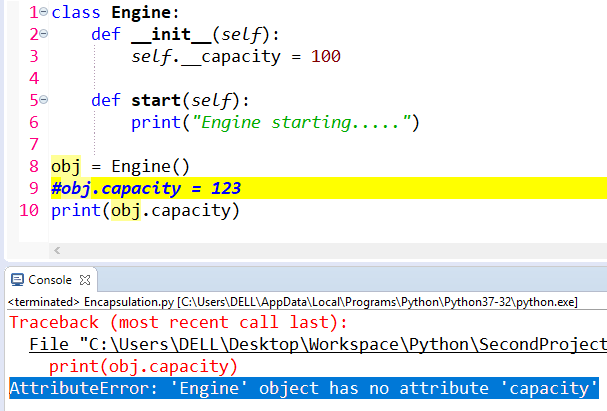Figure 4

Even if we use double underscore (see line 10 below), still gives us the same error
“AttributeError: 'Engine' object has no attribute '__capacity'”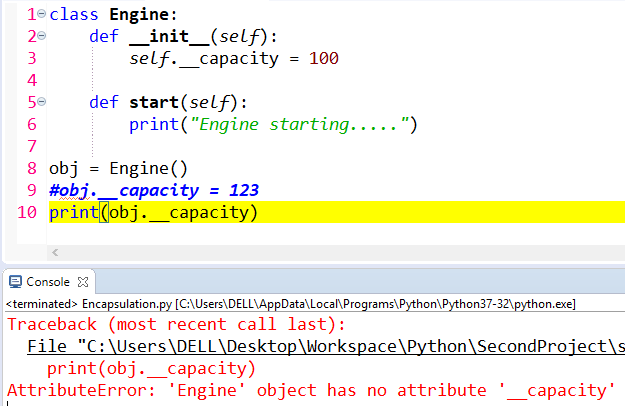Figure 5

If you see line number below, we have changed the value of private variable & can print it as well. So, private variables in python are kind of partial private variables, because we are still able to change them outside the class (line 9)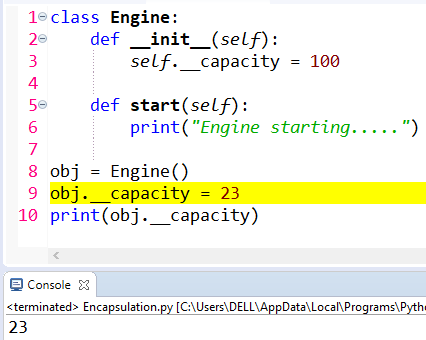Figure 6

Getters
If you would like to print the actual capacity of the engine (value in line 3 below), than we can write a getter function (lines 8-10). We can than call this getter (line 15). When we run program, we see 100 getting printed in console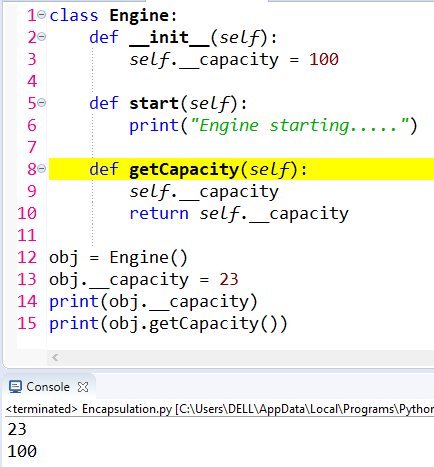Figure 7: getter

Class variable

Let us define a class ‘Car’ (line 17 below) & create a class variable ‘wheels’ (line 18). Class variables are variables that belong to a class.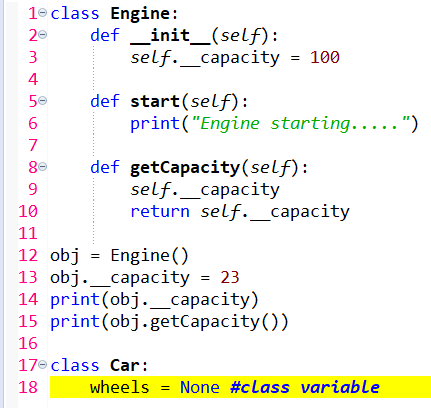Figure 8

Create object inside the class
So far we have been creating objects outside a class. Let us now create an object inside the class. See line 19 below. We created object of ‘Engine’ class inside the ‘Car’ class.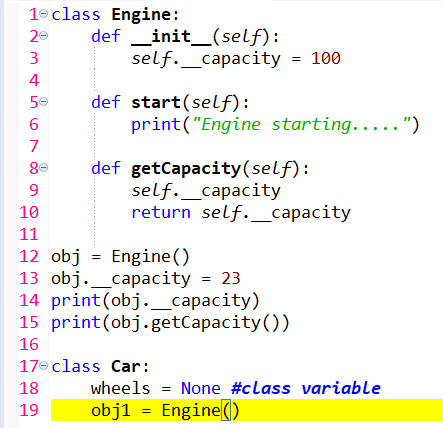Figure 9

We next define the ‘start’ method inside the ‘Car’ class, see line 20 below. We than call the ‘start’ method of ‘Engine’ class (line 21). So when we call this ‘Car’ class, it will automatically start the engine (lines 20-21)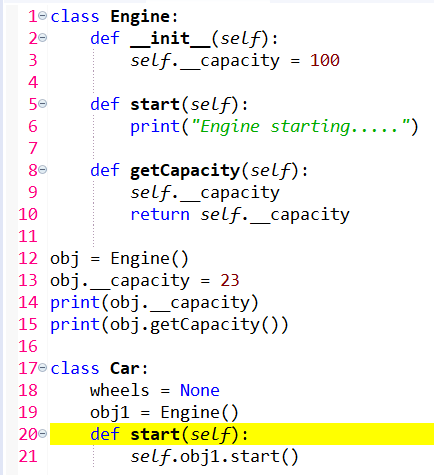Figure 10

So, so far we have 2 classes: Engine class & Car class, see below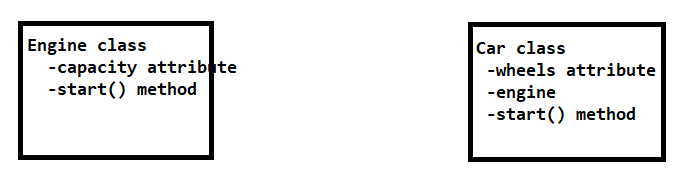Figure 11

Setters
To set the capacity of car, we create another function ‘setCapacity’ (lines 20-22 below)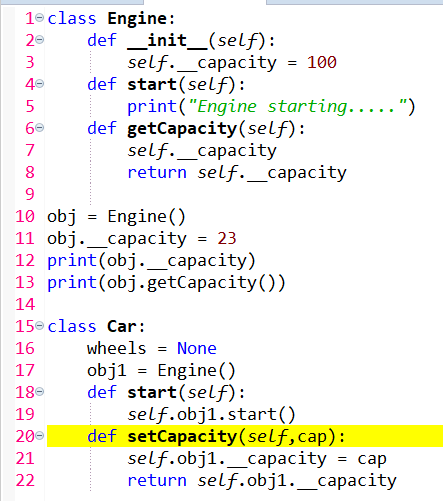Figure 12

Now to set or change the capacity of ‘Car’, we create an object of ‘Car’ class & call the setCapacity function, see below lines 21 & 22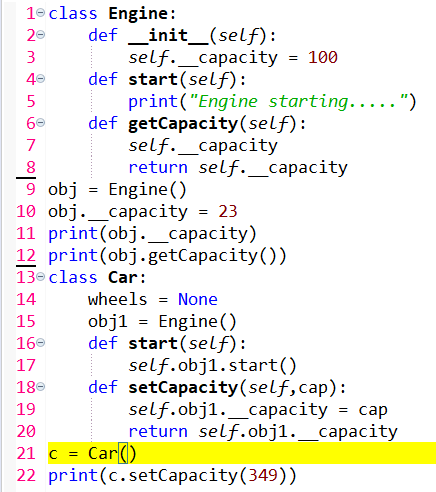Figure 13

When we run, the capacity 349 is printed, see below. So we change the scope of the variable outside the class tooFigure 14

Conclusion
In this tutorial we have studied about public, private variables, getters & setters in python. So in summary, encapsulation is the packing of data and functions operating on that data into a single component and restricting the access to some of the object's components. Encapsulation means that the internal representation of an object is generally hidden from view outside of the object's definition. We will continue with part 2 of encapsulation viz private functions & abstract classes in next tutorial. Thank you for reading!